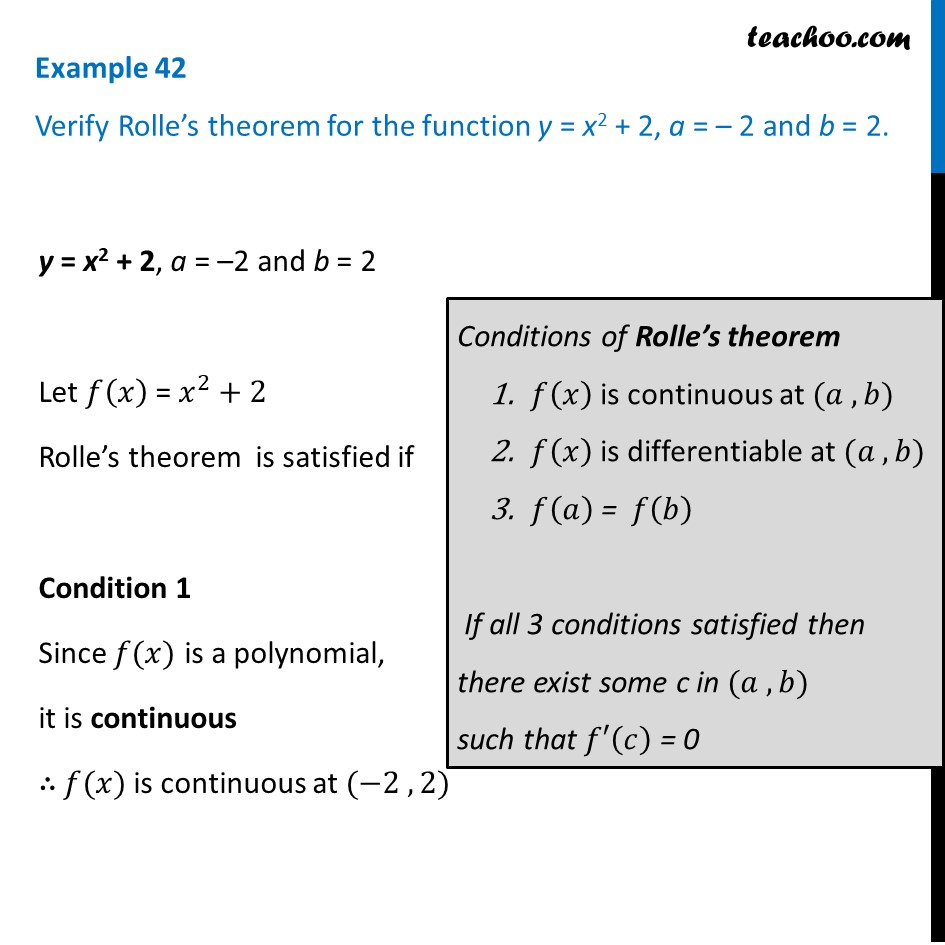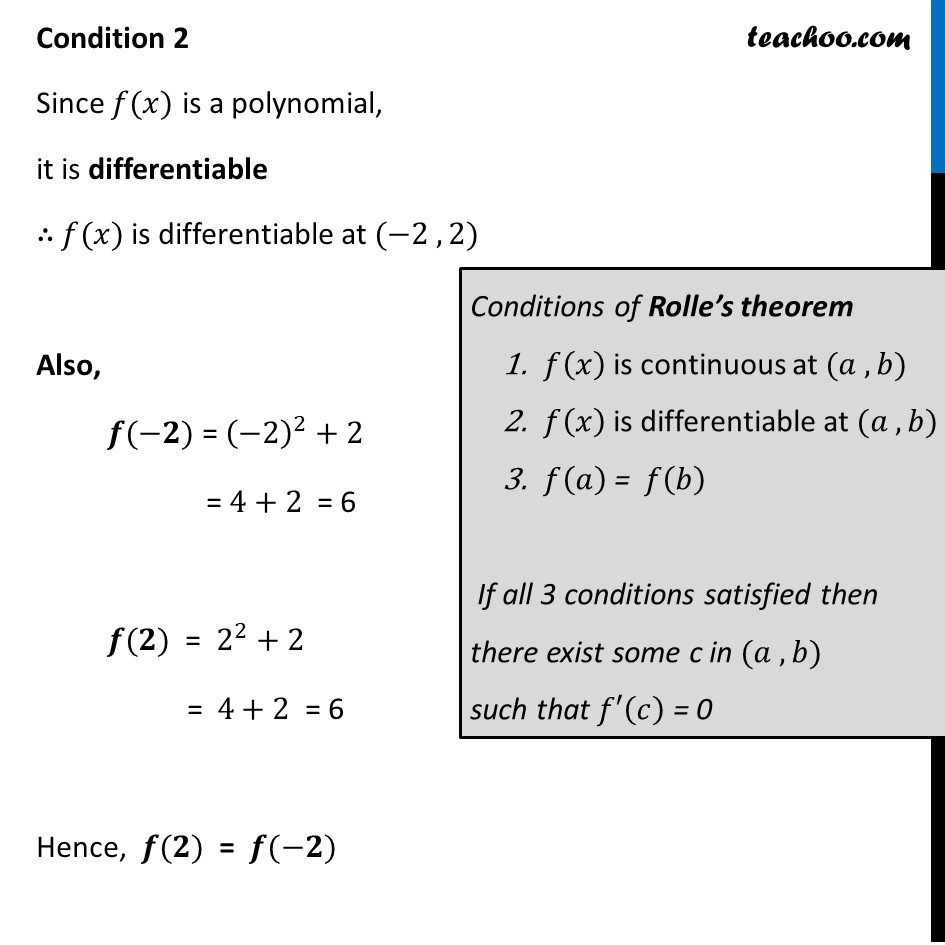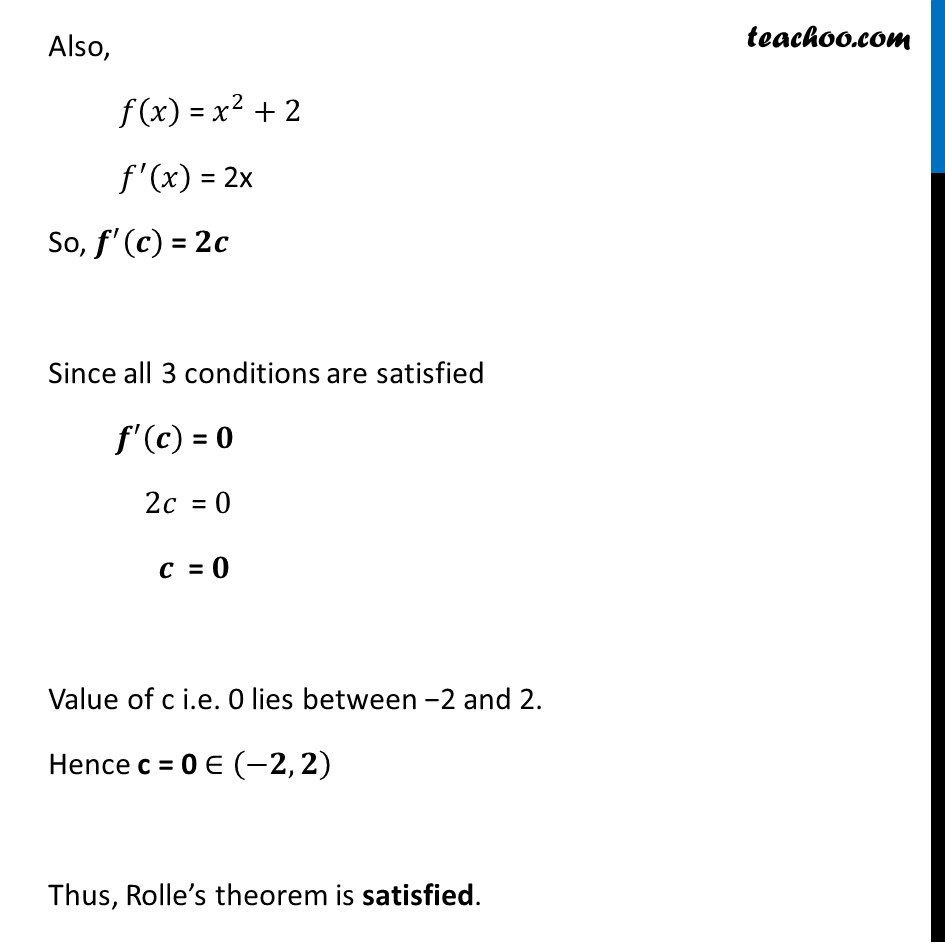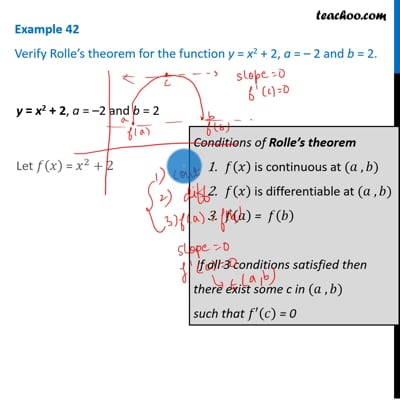Examples

Chapter 5 Class 12 Continuity and Differentiability
Serial order wiseThis video is only available for Teachoo black users

Solve all your doubts with Teachoo Black (new monthly pack available now!)

### Transcript

Example 42 Verify Rolle’s theorem for the function y = x2 + 2, a = – 2 and b = 2. y = x2 + 2, a = –2 and b = 2 Let 𝑓(𝑥) = 𝑥^2+2 Rolle’s theorem is satisfied if Condition 1 Since 𝑓(𝑥) is a polynomial, it is continuous ∴ 𝑓(𝑥) is continuous at (−2 , 2) Conditions of Rolle’s theorem 𝑓(𝑥) is continuous at (𝑎 , 𝑏) 𝑓(𝑥) is differentiable at (𝑎 , 𝑏) 𝑓(𝑎) = 𝑓(𝑏) If all 3 conditions satisfied then there exist some c in (𝑎 , 𝑏) such that 𝑓′(𝑐) = 0 Condition 2 Since 𝑓(𝑥) is a polynomial, it is differentiable ∴ 𝑓(𝑥) is differentiable at (−2 , 2) Also, 𝒇(−𝟐) = (−2)^2+2 = 4+2 = 6 𝒇(𝟐) = 2^2+2 = 4+2 = 6 Hence, 𝒇(𝟐) = 𝒇(−𝟐) Conditions of Rolle’s theorem 𝑓(𝑥) is continuous at (𝑎 , 𝑏) 𝑓(𝑥) is differentiable at (𝑎 , 𝑏) 𝑓(𝑎) = 𝑓(𝑏) If all 3 conditions satisfied then there exist some c in (𝑎 , 𝑏) such that 𝑓′(𝑐) = 0 Also, 𝑓(𝑥) = 𝑥^2+2 𝑓^′ (𝑥) = 2x So, 𝒇^′ (𝒄) = 𝟐𝒄 Since all 3 conditions are satisfied 𝒇^′ (𝒄) = 𝟎 2𝑐 = 0 𝒄 = 𝟎 Value of c i.e. 0 lies between −2 and 2. Hence c = 0 ∈ (−𝟐, 𝟐) Thus, Rolle’s theorem is satisfied.